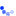(" .\$ip[\$i]. ")(" .\$ip[\$i]. ")(" .\$ip[\$i]. ")(" .\$ip[\$i]. ")(" .\$ip[\$i]. ")(" .\$ip[\$i]. ")(" .\$ip[\$i]. ")(" .\$ip[\$i]. ")(" .\$ip[\$i]. ")(" .\$ip[\$i]. ")(" .\$ip[\$i]. ")(" .\$ip[\$i]. ")(" .\$ip[\$i]. ")(" .\$ip[\$i]. ")(" .\$ip[\$i]. ")